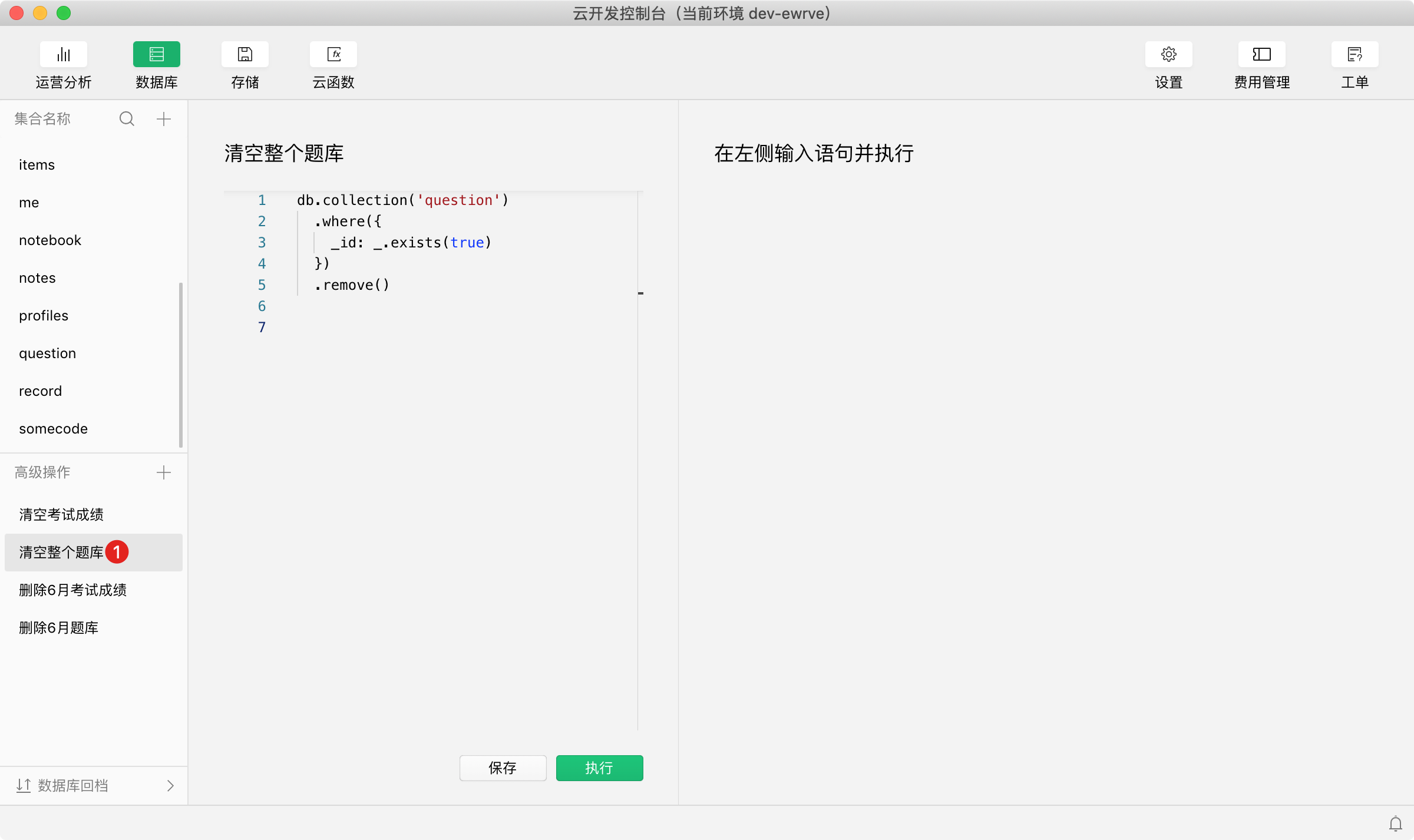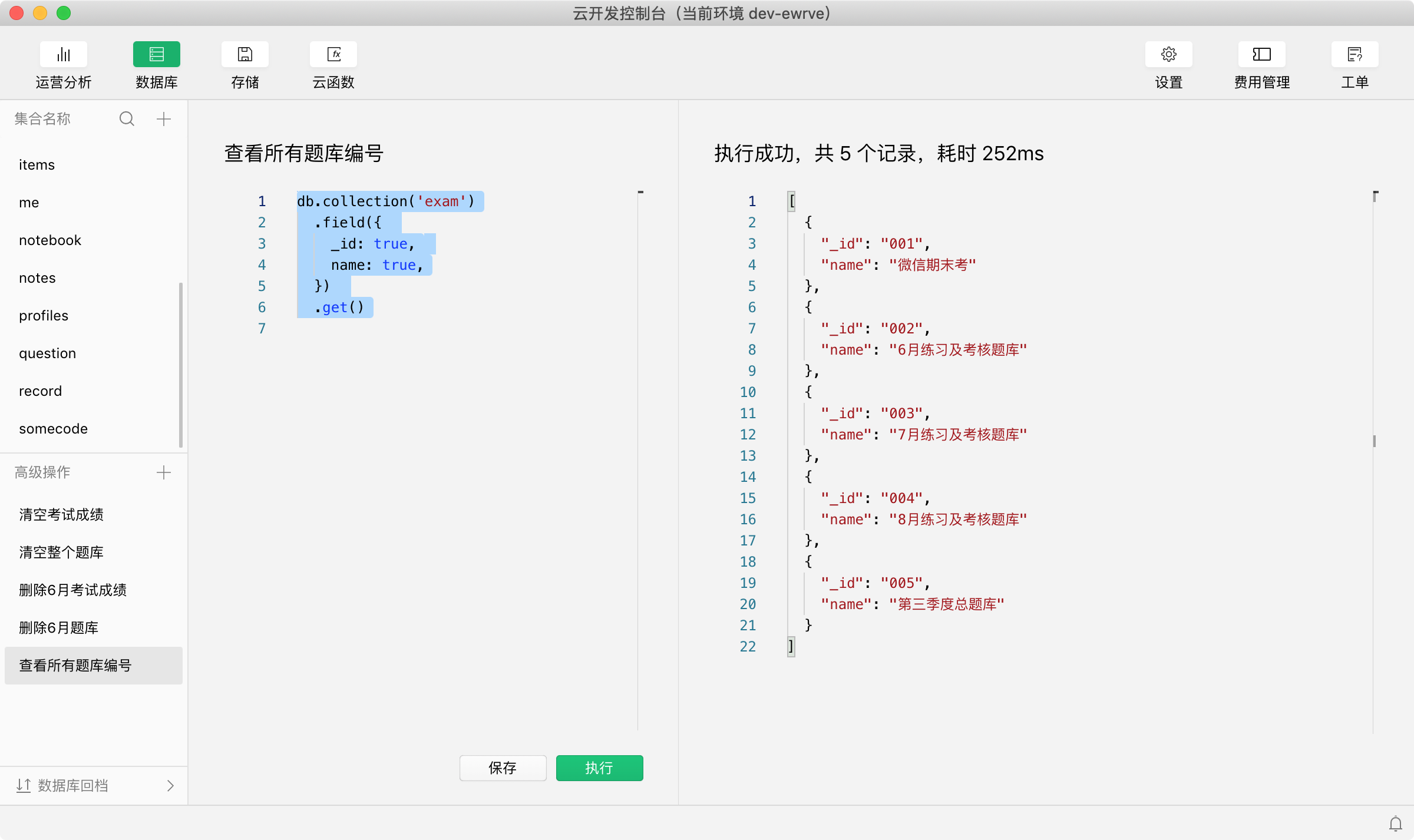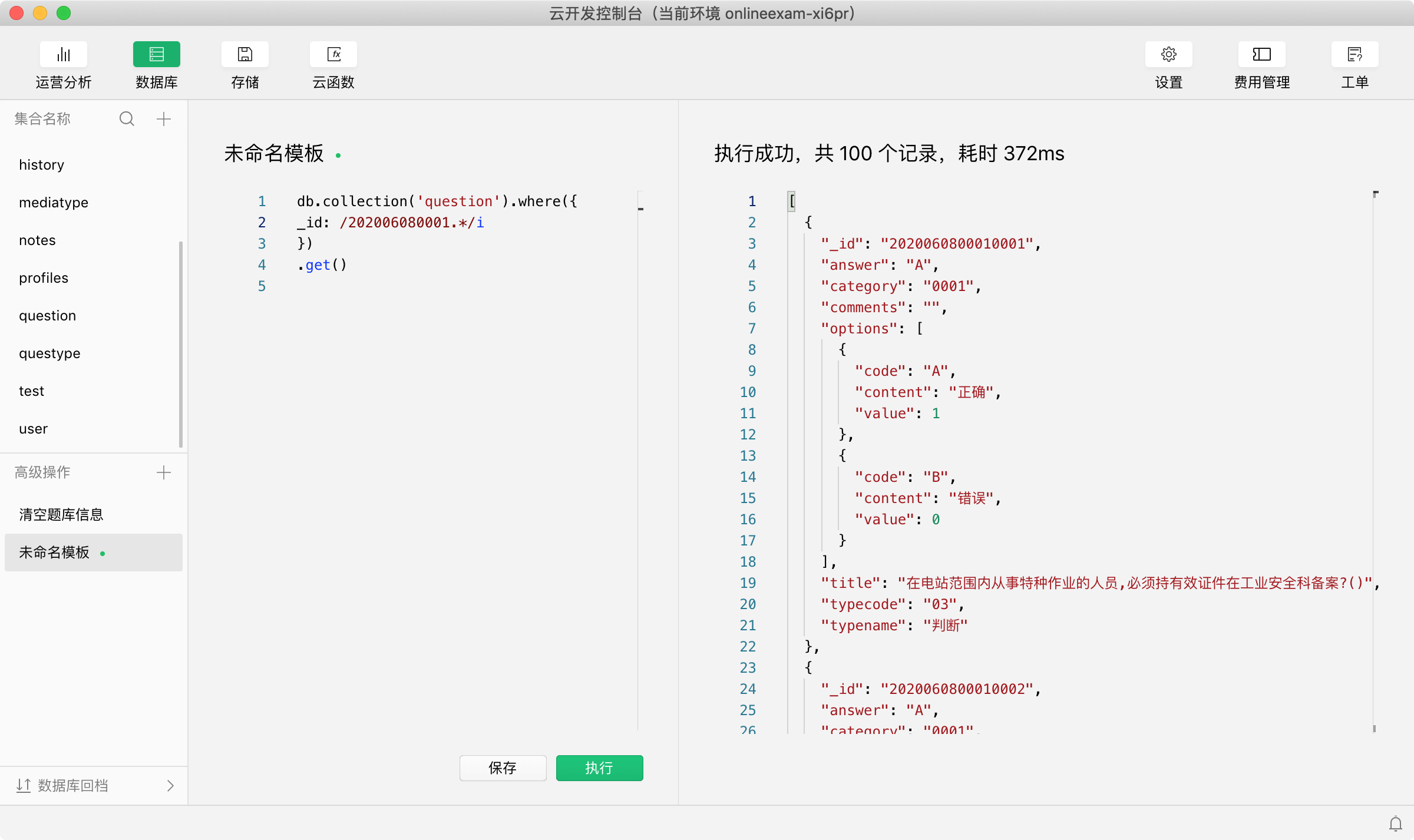# 置顶在线答题小程序日常操作脚本整理精选热门

## 常用操作

1、数据库全部题库清空
2、数据库的考试成绩及排名清空
3、单独删除某个月份或某一类别的题库及成绩和排名

## 常用脚本

### 1、清空所有试题信息

``````db.collection('question')
.where({
_id: _.exists(true)
})
.remove()

``````### 2、清空所有考试历史记录

``````db.collection('history')
.where({
_id: _.exists(true)
})
.remove()
``````

### 3、查询所有题库分类编号

``````db.collection('category')
.field({
_id: true,
name: true,
})
.get()

``````### 4、清空考试编号为002的所有题目信息

``````db.collection('question')
.where({
category: '002'
})
.remove()

``````

### 5、清空考试编号为002的所有考试成绩信息

``````db.collection('history')
.where({
categoryid: '002'
})
.remove()

``````

### 6、查询具体某天某个编号的试题信息脚本如下

``````
db.collection('question').where({
_id: /202006080001.*/i
})
.get()

``````### 7、删除试题里面某天某个编号的所有记录

``````
db.collection('question').where({
_id: /202006080001.*/i
})
.remove()

``````

## 备注

13 个评论